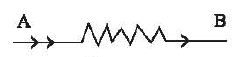# Electric Energy Power

When a potential difference is applied between the two terminals of a conductor, an electric current is set up. Due to this, work is done and the electrons acquire energy. This electrical energy may be transformed into different forms of energy (e.g.- heat, light, mechanical energy etc.) according to the nature of the circuit.Say, AB is a conductor of resistance R and Q amount of charge is flowing through it. The potential difference between the points A and B is V. We know, if the potential difference between the two terminals of a conductor is 1 volt and 1 coulomb charge flows through it, then the amount of work done or energy spent is joule. Now, if Q coulomb charge flows through the conductor, the amount of work done = VQ joule. Therefore, energy spent or the amount of energy converted is W = VQ

Again, Electric Current, I = Q/t

Or, Q = It

So, W = VIt

Using ohm’s law this relationship can be expressed as below,

W = VIt = I2Rt = (V2/R)t Joule